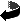# Weighted Sobolev spaces and regularity for polyhedral domains by Bernd Ammann, Victor Nistor

Weighted Sobolev spaces and regularity for polyhedral domains (.dvi, .ps,.ps.gz or .pdf)
Comput. Methods Appl. Mech. Engrg. 196 3650-3659 (2007)
DOI: 10.1016/j.cma.2006.10.022

### Abstract

We prove a regularity result for the Poisson problem \$-\Delta u = f\$, \$u \vert_{\pa \PP} = g\$ on a polyhedral domain \$\PP \subset \RR^3\$ using the \BK\ spaces \$\Kond{m}{a}(\PP)\$. These are weighted Sobolev spaces in which the weight is given by the distance to the set of edges. In particular, we show that there is no loss of \$\Kond{m}{a}\$--regularity for solutions of strongly elliptic systems with smooth coefficients. We also establish a ``trace theorem'' for the restriction to the boundary of the functions in \$\Kond{m}{a}(\PP)\$.Zurück zur Homepage

The Paper was written on 1.12.2005
Last update 9.6.2006